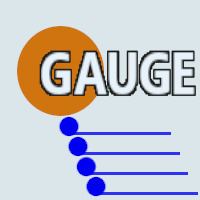# Gauge Lightweight Object

#### @basterdDEV

You must be logged in to obtain assets

### Description

A simple Gms 2.3 script that contains a struct. The struct contains 4 variables and a plethora of static methods. It's designed primarily to represent attributes(common and derived) in RPGs.

Usage: //initialisation //It takes 3 arguments of the real type to initialise the first 3 variables(low_bound,high_bound,value). //If it's not provided an argument of the real type, the corresponding variable is initialized with zero. gauge_struct_variable = new global.Gauge(_value, _low_bound, _high_bound);

//release delete gauge_struct_variable;

Variables: - low_bound => minimum gauge value, the variable value shouldn't be lower than that. - high_bound => maximum gauge value, the variable value shouldn't be higher than that. - value => the actual gauge value, it should be between the low_bound and high_bound closed range. - value_modifier => Its use is more optional than the other variables. This variable mainly represents the sum of all active bonus/malus modifiers on an attribute.

Static Methods: - Fix_Gauge() => force the low_bound < high_bound rule and keep the gauge value in the [low_bound, high_bound] range. - Set_value(real) => assigns a new value to the gauge value and calls the Fix_Gauge() static method. If the passed argument isn't a real type, it won't modify the gauge value nor fix the gauge.
- Set_low_bound(real) => assigns a new value to the gauge low_bound and calls the Fix_Gauge() static method. If the passed argument isn't a real type, it won't modify the gauge low_bound nor fix the gauge.
- Set_high_bound(real) => assigns a new value to the gauge high_bound and calls the Fix_Gauge() static method. If the passed argument isn't a real type, it won't modify the gauge high_bound nor fix the gauge. - Add_to_value(real) => add a value directly to the gauge value and calls the Fix_Gauge() static method. If the passed argument isn't a real type, it won't modify the gauge value nor fix the gauge.
- Add_to_low_bound(real) => add a value directly to the gauge low_bound and calls the Fix_Gauge() static method. If the passed argument isn't a real type, it won't modify the gauge low_bound nor fix the gauge.
- Add_to_high_bound(real) =>add a value directly to the gauge high_bound and calls the Fix_Gauge() static method. If the passed argument isn't a real type, it won't modify the gauge high_bound nor fix the gauge. - Approach_to_low_bound(real) => approach the minimum boundary by subtracting an absolute value directly to the gauge value. If the gauge value is greater than the maximum boundary; the gauge value will be set on that value, before proceeding with the approach operation. If the passed argument isn't a real type, it won't modify the gauge value nor fix the gauge. - Approach_to_high_bound(real) => approach the maximum boundary by adding an absolute value directly to the gauge value. If the gauge value is lesser than the minimum boundary; the gauge value will be set on that value, before proceeding with the approach operation. If the passed argument isn't a real type, it won't modify the gauge value nor fix the gauge.

• Get_DnD_ability_modifier_from_value() => get an Dungeons and Dragons style ability score modifier from the gauge value.
• Get_DnD_ability_modifier_from_raw_value() => get an Dungeons and Dragons style ability score modifier based on the gauge raw value(value + value_modifier).
• Get_custom_ability_modifier_from_value(real,real) => get an DnD inspired rpg ability score modifier from the gauge value. Return undefined if any of the arguments passed type isn't real type.
• Get_custom_ability_modifier_from_raw_value(real,real) => same behaviour as above, but using the the gauge raw value instead.
• To_Min() => set the gauge value with the smallest value between the low_bound and high_bound.
• To_Max() => set the gauge value with the biggest value between the low_bound and high_bound.
• Get_raw_value() => get the value of the sum betwneen the gauge value and the value modifier.